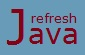# Java programs of control flow statements

This tutorial shows some of the useful programs which are generally asked with beginners. These programs are using control flow statements in some-way or other. These programs will definitely help them to build different logics for different types of programs. Let's see the first program which shows how to find if a number is even or odd.

### Java Program of even odd number

```` class` EvenOddProgram
{
`public static void` main(String [] args)
{
`int` num1 = 22;
`if`(num1 % 2 == 0)
{
System.out.println(num1+ `" is an even number"`);
}
`else`
{
System.out.println(num1+ `" is an odd number"`);
}
}
}```

Output :

22 is an even number

`%` returns remainder. We know that if a number is divisible by 2, it's an even number. Since 22 is divisible by 2, as it returns remainder as 0 which means 22 is an even number. Just change the `num1` as `23`, the output will be "`23 is an odd number`."

Sometime as a beginner you might be ask to write a program which prints multiplication table of a number. Program below shows how to print multiplication table of a given number, just change the `num1` as any other integer number, the program will display the table of that number.

### Java Program to print multiplication table of a number

```` class` NumberTable
{
`public static void` main(String [] args)
{
`int` num1 = 8;
`for`(`int` i=1; i<=10;i++)
{
System.out.println(num1*i);
}
}
}```

Output :

8
16
24
32
40
48
56
64
72
80

Sometime programmers are asked to write a program to find the greatest of three number, program below shows how to find the greatest of three number. you can change the value of `num1`, `num2`, `num3` the program will print the greatest of all three numbers. This is one approach to find the greatest number, there could be other approaches as well.

### Java Program to find greatest of three number

```` class` GreatestOfThreeNumber
{
`public static void` main(String [] args)
{
`int` num1 = 40, num2 = 20, num3 = 50;
`if`(num1 > num2)
{
`if`(num1 > num3)
System.out.println(`"Greatest number = "`+num1);
`else`
System.out.println(`"Greatest number = "`+num3);
}
`else`
{
`if`(num2 > num3)
System.out.println(`"Greatest number = "`+num2);
`else`
System.out.println("`Greatest number = "`+num3);
}
}
}```

Output :

Greatest number = 50

Let's write one more program which shows how to find the grade on the basis of marks provided. You can change the marks, the program will display the appropriate grade.

### Java Program of finding grade

```` class` FindingGrade
{
`public static void` main(String [] args)
{
`int` marks = 65;
`if`(marks >= 80)
System.out.println(`"A+ grade"`);
`else if`(marks >= 60 && marks < 80)
System.out.println(`"A grade"`);
`else if`(marks >= 40 && marks < 60)
System.out.println(`"B grade"`);
`else if`(marks >= 30 && marks < 40)
System.out.println(`"C grade"`);
`else`
System.out.println(`"D grade"`);
}
}```

Output :